## Sunday, 15 June 2008

### Excel area chart with colour invert if negative

My intention is for this blog to be commentary on graphs and data, rather than instructions on drawing graphs using any particular program. But I can make an exception, and here's a technique I've worked out for making Area charts in Excel that change colour below the zero line. This is something that comes as a standard option in bar charts. consider the following table:This makes a bar chart okay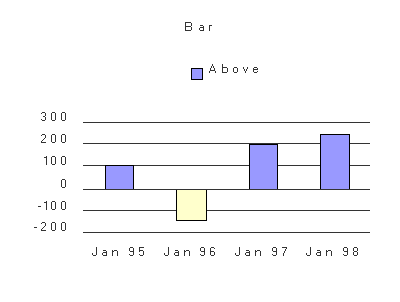But not such a good area chart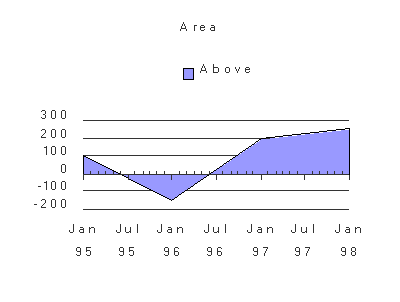We can create a table that splits the positive and negative valuesbut the result is disappointing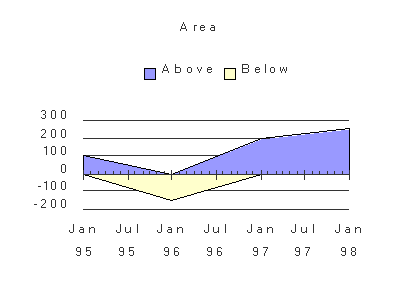The areas simply go to zero at the next value, which is not what we want. This happens even when the Excel Time-scale X axis type is selected by going to Chart..Chart Options..Axes and selecting "Time-scale". However, Time-scale has one feature that lets us easily fix the problem. Unlike the ordinary category axis, it does not present values in the same order as they appear in the table, but in strict time order. So if we make a new set of rows below our first set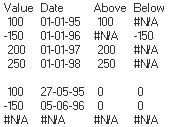where the formulae are (left to right, assuming the original table header started at cell A1)
`=IF(\$A2*\$A3>0,NA(),\$A2)=IF(\$A2*\$A3>0,NA(),\$B2+(\$B3-\$B2)*\$A2/(\$A2-\$A3))=IF(\$A2*\$A3>0,NA(),0)=IF(\$A2*\$A3>0,NA(),0)`

then the two areas should meet the zero line at the same interpolated date!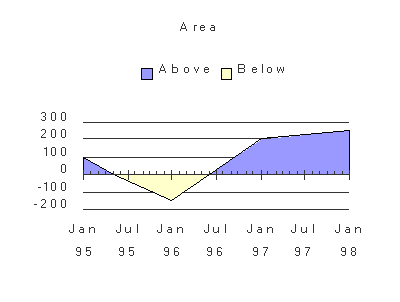Remember, this only works if you've selected the "Time-scale" option in Chart Options. If you're looking for more Excel help, see the Jon Peltier and Andy Pope links on the right.